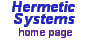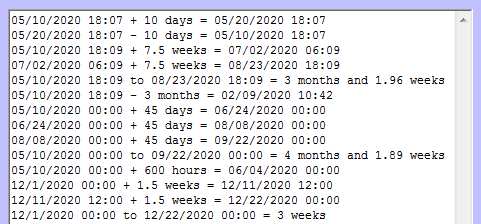# Date-Time Calculator## Software to perform conversion, addition and subtraction of dates and times.

Here is a typical screenshot.If you want a record of operations performed then click on the Show results button, which reveals an output window. Here is an example:The results of operations are recorded in the output window only when it is open.

The various settings can be saved and restored using the Save/Restore buttons. The settings at the last run are restored automatically at the next start up.

Operating Instructions

First select your preferred date/time format: year‑month‑day, month/day/year or day.month.year. The date must be a date in the Gregorian (a.k.a. Common Era) Calendar. Then there are four date/time operations which can be performed:

1. Convert a period of time stated with some time unit to that period in other units.
• Select a time unit: minutes, hours, days, etc.
• Specify the number of hours, days, etc. in the Time period box.
• Click on the Convert time period button.
• That period of time will then be displayed in the boxes for Minutes, etc.
This operation does not require specification of a start or an end date/time. A number of weekdays is not shown because that depends on start and end dates.

2. Add a period of time to the start date/time.
• Enter the date and time in the start date/time boxes.
• Select a time unit: minutes, hours, days, etc.
• Specify the number of hours, days, etc. in the Time period box that you wish to add to the start date/time.
• Specify the number of times you want this addition to be performed successively.
• Click on the Add time period button.
• The end time will then be shown in the end date/time boxes
Note that the period of time ends with the end time at the end date. If the end time is 00:00 then none of the day of the end date is included in the time period. Thus if both times are 00:00 then the number of days (and the number of weekdays) will not include the day of the end date.
Note also that if the addition is to be performed more than once then the end time is that which results from performing all additions successively. In the example shown in the screenshot, each step is 3 weeks, but since the addition was done twice the end date/time box shows the result of adding 6 weeks to the start date/time.

3. Subtract a period of time from the start date/time.
• Enter the date and time in the start date/time boxes.
• Select a time unit: minutes, hours, days, etc.
• Specify the number of hours, days, etc. in the Time period box that you wish to subtract from the start date/time.
• Specify the number of times you want this subtraction to be performed successively.
• Click on the Subtract time period button.
• The end time will then be shown in the end date/time boxes.
Since time does not run backwards, when subtracting note that the actual period of time begins with the end date/time and ends with the start date/time. If the start time is 00:00 then none of the day of the start date is included in the time period.

4. Get the period of time from the start date/time to the end date/time
• Enter the start date/time and the end date/time in the boxes (or click on Now to use your computer's date/time as the start date/time).
• If you want to get the number of weekdays in the time period then click on the Midnight button.
• Click on the Time period from ... button.
• The time period will then be shown in Time period box and in the boxes below (in the various time units).
As stated above, note that the period of time ends with the end time at the end date. When both times are 00:00 then the number of weekdays will not include the day of the end date.
Note also that you do not need to first add a time period to the start date — the operation will act on any start date/time and end date/time (provided the latter is after the former).

Trial version: A copy of Date-Time Calculator is available for free download from this website for the purpose of evaluation. Click on the following link for further information: